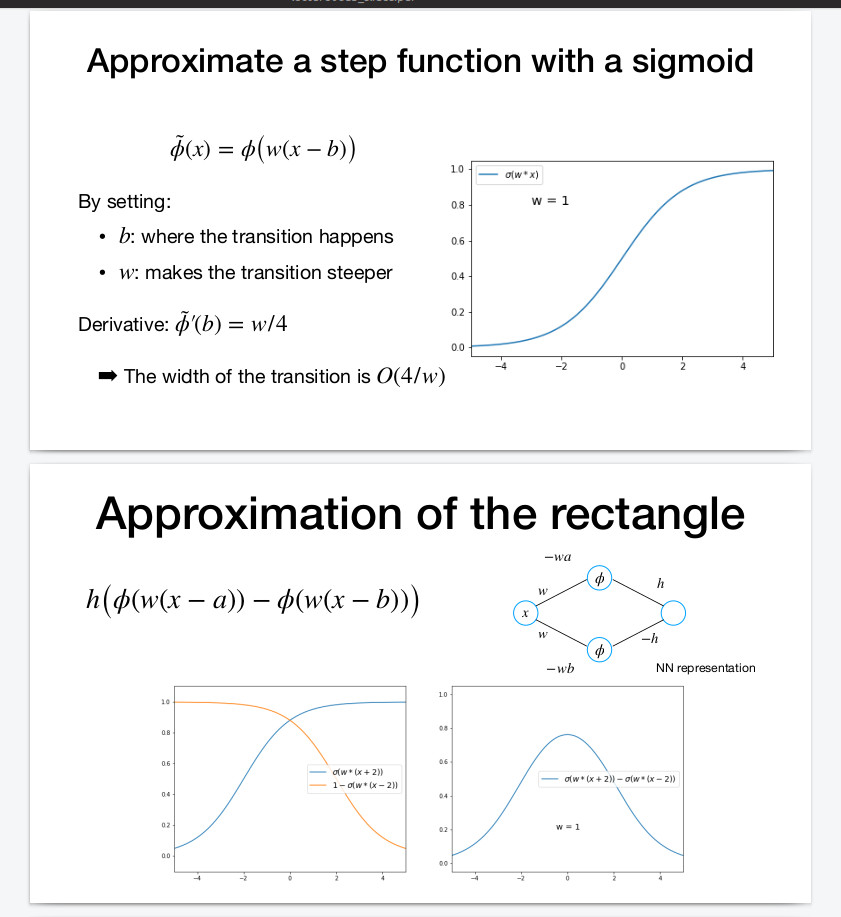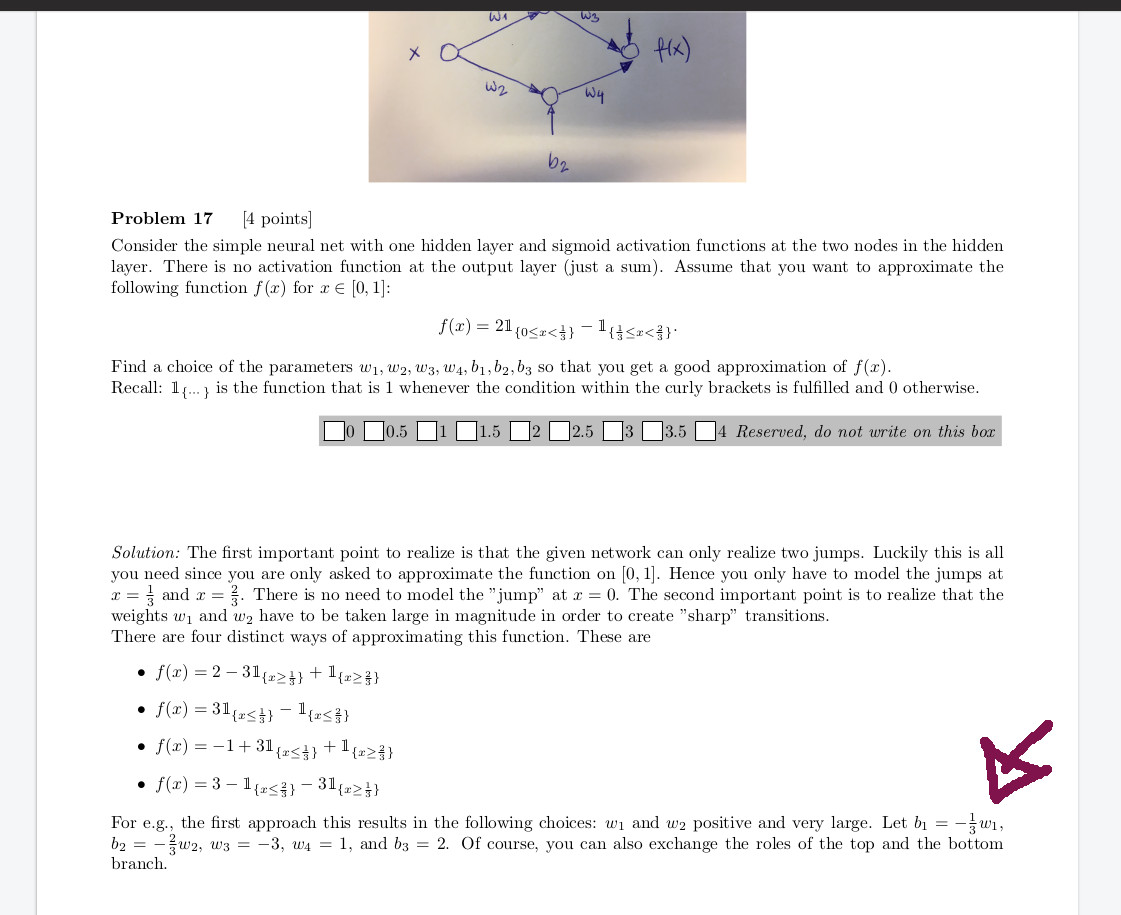### Sigmoid Fonction transition for Neural Network

Hello

In this lectures said that b is where the transition happen.
But on the Neural network we add a coefficient : -wb :
With the correction on Open question 17 from examen 2018 , we see that for a transition to happen at 1/3 , it set b = -1/3
w.

So where the transition happen on a 1-D neural network ? b or -w*b ?Top comment

I think "b is where the transition happen" means we adjust b to shift the decision boundary, while in theoretical analysis, b is actually proportional to w if you want to get a integer solution, because x is scaled by w

Page 1 of 1